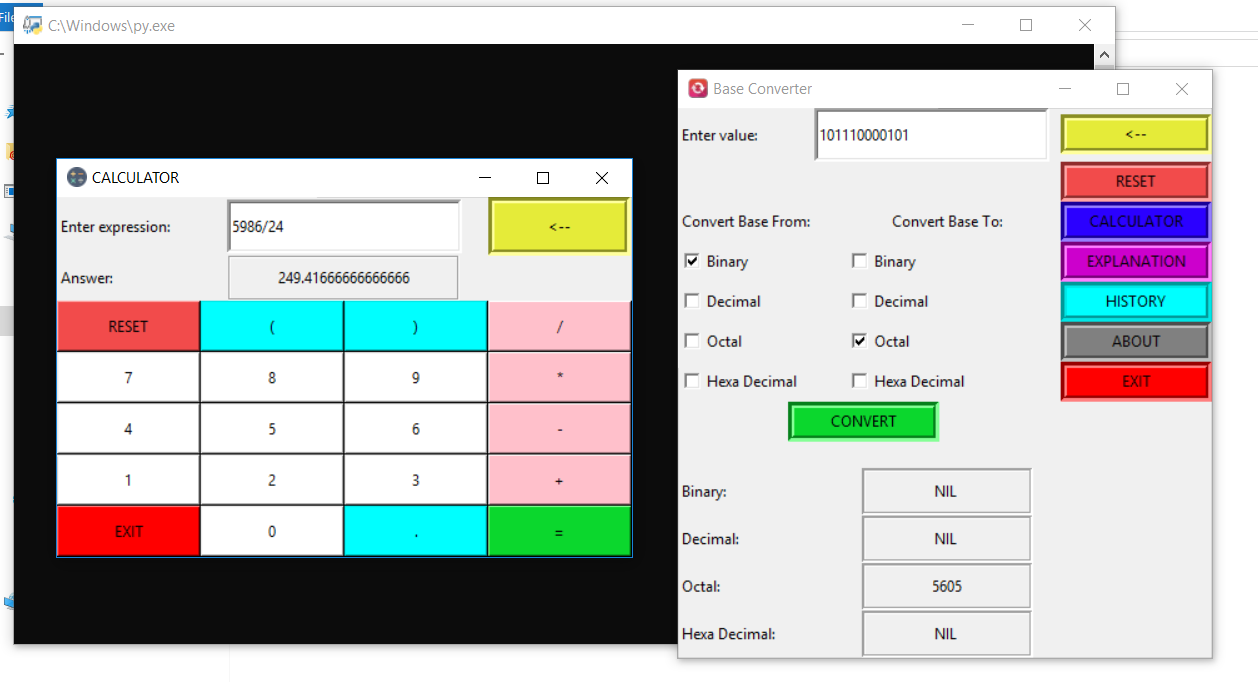## BASE CONVERTER APP IN PYTHON WITH SOURCE CODE

The Base converter App In Python is a simple project developed using Python. The project contains a base conversion system and calculator. The user can enter any number and convert them to any number systems(like decimal to binary, binary to octal, etc.).

Type : Project

File Size : 71.9 KB# Similar Projects and Reports

#### BASE CONVERTER APP IN PYTHON WITH SOURCE CODE

The Base converter App In Python is a simple project developed using Python. The project contains a base conversion system and calculator. The user can enter any number and convert them to any number systems(like decimal to binary, binary to octal, etc.).

#### ALL IN ONE CONVERTER APP IN PYTHON WITH SOURCE CODE

The All In One converter App In Python is a simple project developed using Python. This project is a GUI application which converts different quantities like Area, Length, Weight, Temperature and Currency from one unit to another. Also, this app is capabl...

#### Numerical Base Converter In Python With Source Code

The Numerical Base converter In Python is a simple project developed using Python. The project contains a base conversion system. The user can enter any number and convert them to any number systems(like decimal to binary, binary to octal, etc.).

#### Base Converter In C++ With Source Code

The Base converter is a simple project developed using c++ programming language. The project contains a base conversion system. The user can enter any number and convert them to any number system (like decimal to binary, binary to Hexadecimal, etc.).

#### Binary converter In Python with Source code

The Binary converter In Python is a simple project developed using Python. The project contains a base conversion system. The user can enter any number and convert them to other number systems (decimal to binary, binary to decimal).

#### Weight Converter In Python With Source Code

The Weight converter In Python is a simple project developed using Python. This project is a GUI application that converts your weight into different units. Here, you have to enter the weight in kg and the result will be in other different units. And, the...